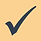top of page

## Topic 9: Calculating with DecimalsIn the calculator section of your exam, you will be asked to add, subtract and multiply decimals. This should be straightforward, as long as you use your calculator carefully and always double check your answers.

But you will also have to perform these calculations in the non-calculator section of the exam.

You can work in columns using the same methods you would use when working with integers.

Here are some examples of decimal calculations laid out in columns.

Example: 3.55 + 17.1 = 20.65

Notice that the empty hundredths column of 17.1 has been marked with a zero, to give both numbers two decimal places.

Subtraction

Example: 12.4 - 5.15 = 7.25

Notice that the empty hundredths column of 12.4 has been marked with a zero, to give both numbers two decimal places.

Multiplication

Example: 9.62 × 5 = 48.1

Division

Example: 70.65 ÷ 5 = 14.13

Notice that for all four operations,a decimal point must be marked in the answer box in the same place as it is marked in the given numbers.

If in doubt about how the numbers should line up, remember that the decimal points must always be in line.bottom of page